# list coloring

## List Colourings of Complete Multipartite Graphs with 2 Big Parts ★★

Author(s): Allagan

Question   Given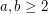, what is the smallest integersuch that?

## List Total Colouring Conjecture ★★

Author(s): Borodin; Kostochka; Woodall

Conjecture   Ifis the total graph of a multigraph, then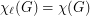.

Keywords: list coloring; Total coloring; total graphs

## Choosability of Graph Powers ★★

Author(s): Noel

Question  (Noel, 2013)   Does there exist a function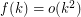such that for every graph,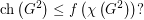## Bounding the on-line choice number in terms of the choice number ★★

Author(s): Zhu

Question   Are there graphs for whichis arbitrarily large?

## Choice number of complete multipartite graphs with parts of size 4 ★

Author(s):

Question   What is the choice number of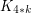for general?

## Choice Number of k-Chromatic Graphs of Bounded Order ★★

Author(s): Noel

Conjecture   Ifis a-chromatic graph on at mostvertices, then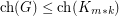.

## Ohba's Conjecture ★★

Author(s): Ohba

Conjecture   If, then.

## Partial List Coloring ★★★

Letbe a simple graph, and for every list assignment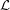letbe the maximum number of vertices ofwhich are colorable with respect to. Define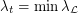, where the minimum is taken over all list assignmentswith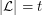for all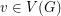.

Conjecture    Letbe a graph with list chromatic numberand. Then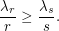Keywords: list assignment; list coloring

## Partial List Coloring ★★★

Author(s): Albertson; Grossman; Haas

Conjecture   Letbe a simple graph withvertices and list chromatic number. Suppose thatand each vertex ofis assigned a list ofcolors. Then at least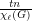vertices ofcan be colored from these lists.

Keywords: list assignment; list coloring

## List colorings of edge-critical graphs ★★

Author(s): Mohar

Conjecture   Suppose thatis a-edge-critical graph. Suppose that for each edgeof, there is a listofcolors. Thenis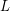-edge-colorable unless all lists are equal to each other.

Keywords: edge-coloring; list coloring